# Test: Inverse Trigonometry -2

## 25 Questions MCQ Test Mathematics (Maths) Class 12 | Test: Inverse Trigonometry -2

Description
This mock test of Test: Inverse Trigonometry -2 for JEE helps you for every JEE entrance exam. This contains 25 Multiple Choice Questions for JEE Test: Inverse Trigonometry -2 (mcq) to study with solutions a complete question bank. The solved questions answers in this Test: Inverse Trigonometry -2 quiz give you a good mix of easy questions and tough questions. JEE students definitely take this Test: Inverse Trigonometry -2 exercise for a better result in the exam. You can find other Test: Inverse Trigonometry -2 extra questions, long questions & short questions for JEE on EduRev as well by searching above.
QUESTION: 1

### cos2θ is not equal to

Solution:

cos 2θ is equal to cos2θ - sin2θ = 2cos2θ - 1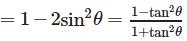QUESTION: 2

###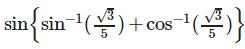is equal to

Solution:

sin-1√3/5 = A
Sin A = √3/5 , cos A = √22/5
Therefore Cos-1√3/5 = B
Cos B = √3/5 , sin B = √22/5
sin(A+B) = sinA cosB + cosA sinB
= √3/5 * √3/5 + √22/5 * √22/5
= 3/25 * 22/25
= 25/25
= 1

QUESTION: 3

### 2cos−1x = cos−1(2x2−1)holds true for all

Solution:

This is true for all real values of x ∈ [0,1].

QUESTION: 4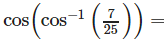Solution:

none of these  : 7/25

QUESTION: 5

If sin A + cos A = 1, then sin 2A is equal to

Solution:

(Sin A + Cos A)2 = sin2A + cos2A + 2 sinAcosA

1 = 1 + Sin 2A

so, Sin 2A = 0

Hence A = 0

QUESTION: 6

When x = π/2, then tan x, is

Solution:

tan π/2 = n.d.i.e. not defined.

QUESTION: 7

The value of the expression sinθ+cosθ lies between

Solution:

Minimum value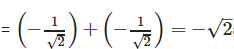and maximum value =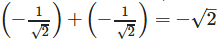QUESTION: 8

cos−1(cosx) = x is satisfied by ,

Solution:

cos−1(cosx) = x if,
0⩽x⩽ π i.e. if , x∈ [0,π]

QUESTION: 9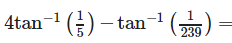Solution: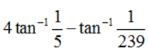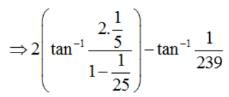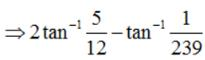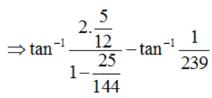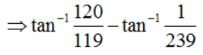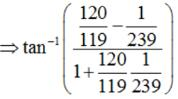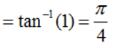QUESTION: 10

If ƒ(x) = tan(x), then f-1(1/√(3)) =

Solution:

Let F(x) = tanx = y
f(-1) = tany = x
f(-1)(1/√3), tany = 1/√3
y = tan-(1/√3)
=π/6

QUESTION: 11

tan x is periodic with period

Solution:

The values of tan x repeats after an interval of π.

QUESTION: 12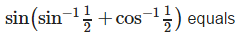Solution: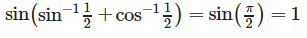QUESTION: 13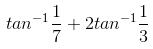is equal to

Solution: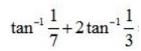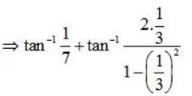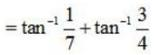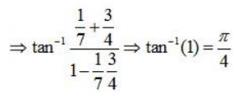QUESTION: 14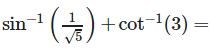Solution: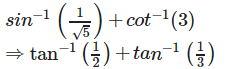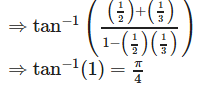QUESTION: 15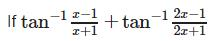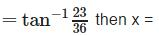Solution:

The correct option is B.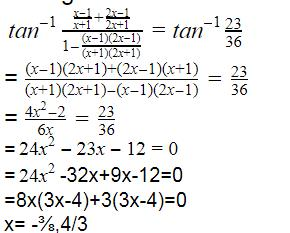QUESTION: 16

The period of the function f(x) = tan 3x is

Solution:

f(x) = tan 3x, as the period of tan x = π. 3x = x = π/3.

QUESTION: 17

The range of tan−1 x is

Solution:

The range of tan−1x is given by -π/2 to π/2

QUESTION: 18

The value of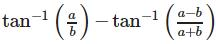is (a, b > 0)

Solution: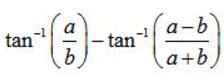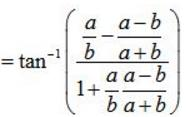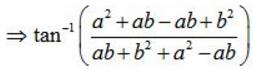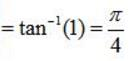QUESTION: 19

The solution of the equation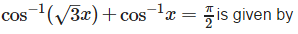Solution: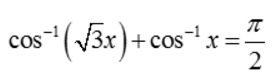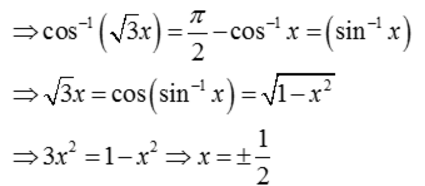But , x= -1/2 does not satisfy the given equation.

QUESTION: 20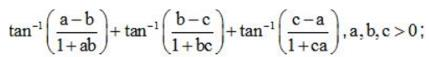is equal to

Solution: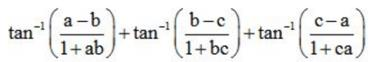= tan-1 a - tan-1 b + tan-1c + tan-1c - tan-1a

QUESTION: 21

sin2250+sin2650 is equal to

Solution:

sin2250 + sin2650 = cos2650 + sin2650 = 1

QUESTION: 22

If θ = tan−1x then sin 2θ is equal to

Solution:

As we know that : sin 2θ =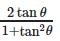, now if  θ = tan-1x ⇒ x = tan θ ⇒ sin 2θ =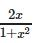QUESTION: 23

if x > 0, then tan-1x + tan-1(1/x) is equal to

Solution: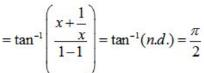QUESTION: 24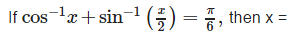Solution: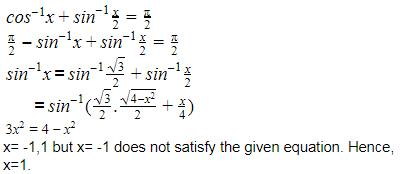QUESTION: 25

If and x + y + z = xyz, then a value of tan−1x+tan−1y+tan−1z is

Solution: